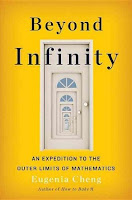## Posts

Showing posts from April, 2023

### Book Review: Beyond InfinityGeeking out is something I yearn to do from time to time. Having seen a documentary on infinity from Netflix last year, I decided to pick up a book on the topic.  Beyond Infinity , by  Eugenia Cheng , is an excellent guide delving into this mind-boggling notion. In this book, Cheng explores some key aspects of infinity that challenge our intuition and understanding of mathematics: Numbers Numbers Numbers, natural, rational, irrational and real:   Cheng explains that these number sets are infinite in nature but some are "more infinite" than others.  For instance, the set of natural numbers are smaller than its superset of rational numbers, which in turn are smaller than the set of real numbers.  So far so good?  But...  Infinity is but an abstract notion: It certainly is not a number   to which the rules of arithmetics apply: ∞ + 1 = ∞ (addition/subtraction does not apply) 2  ⋅  ∞ = ∞ (neither does multiplication/division) 1/∞ = 0 (in the sense of limits which touches on the f